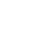# Python中的15个内置字符串方法capitalize()

``>>> txt = "the python is good!">>> txt.capitalize()'The python is good!'``

upper()

``>>> txt = "the python is good!">>> txt.upper()'THE PYTHON IS GOOD!'``

lower()

``>>> txt = "THE PYTHON IS GOOD!">>> txt.lower()'the python is good!'``

isupper()

``>>> txt = "THE PYTHON IS GOOD!">>> txt.isupper()True``

islower()

``>>> txt = "THE PYTHON IS GOOD!">>> txt.islower()False``

isnumeric()

``>>> txt = "THE PYTHON IS GOOD!">>> txt.isnumeric()False>>> txt = "123">>> txt.isnumeric()True``

isalpha()

``>>> txt = "PYTHON">>> txt.isalpha()True>>> txt = "PYTHON123">>> txt.isalpha()False``

isalnum()

``>>> txt = "PYTHON123">>> txt.isalnum()True>>> txt = "PYTHON123!">>> txt.isalnum()False``

count()

``>>> txt = "THE PYTHON IS GOOD!">>> txt.count("T")2``

find()

``>>> txt = "THE PYTHON IS GOOD!">>> txt.find("P")4``

``>>> txt = "THE PYTHON IS GOOD!">>> txt.find("T",2)6``

startswith()

``>>> txt = "PYTHON123!">>> txt.startswith("P")True``

endswith()

``>>> txt = "PYTHON123!">>> txt.endswith("!")True``

replace()

``>>> txt = "PYTHON123!">>>  txt.replace("123", " is good")'PYTHON is good!'``

split()

``>>> txt = "THE PYTHON IS GOOD!">>> txt.split()['THE', 'PYTHON', 'IS', 'GOOD!']``

partition()

``>>> txt = "THE PYTHON IS GOOD!">>> txt.partition("IS")('THE PYTHON ', 'IS', ' GOOD!')``

《Python中的15个内置字符串方法》